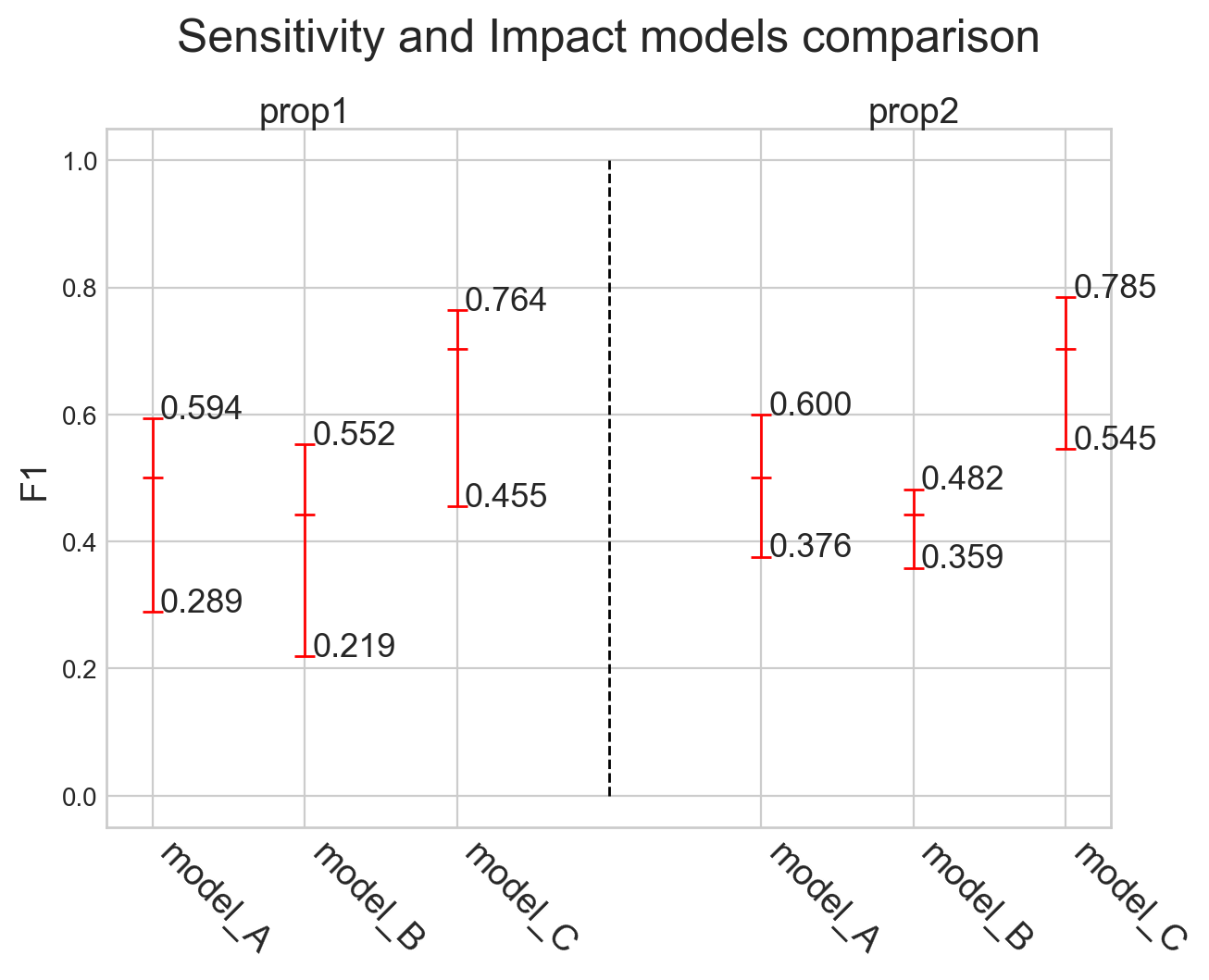# analyze_property()

The model performances are analyzed for each category considering subsets of the data set which have a specific property value.

#### Parameters

property_name

str
Name of the property to be analyzed.
possible_values

list, optional
Property values to be analyzed. If not specified, all the property values are considered.
(default is None)
categories

list, optional
List of categories to be included in the analysis. If not specified, all the categories are included.
(default is None)
show

bool, optional
Indicates whether the plot should be shown or not. If False, returns the results as dict.
(default is True)
metric

Metrics, optional
Evaluation metric used for the analysis. If not specified, the default one is used.
(default is None)
split_by

str, optional
If there are too many values, the plot is divided into subplots. The split can be performed by 'categories' or by 'meta-annotations'.
(default is 'meta-annotations')
sort

bool, optional
Indicates whether the property values should be sorted by the score achieved. Not supported for models comparisons.
(default is True)

### Example

#### Classification

``````from odin.classes import AnalyzerClassification

my_analyzer = AnalyzerClassification("my_classifier_name", my_classification_dataset)
my_analyzer.analyze_property('prop1')
``````

#### Localization

``````from odin.classes import AnalyzerLocalization

my_analyzer = AnalyzerLocalization("my_detector_name", my_localization_dataset)
my_analyzer.analyze_property('prop1')
``````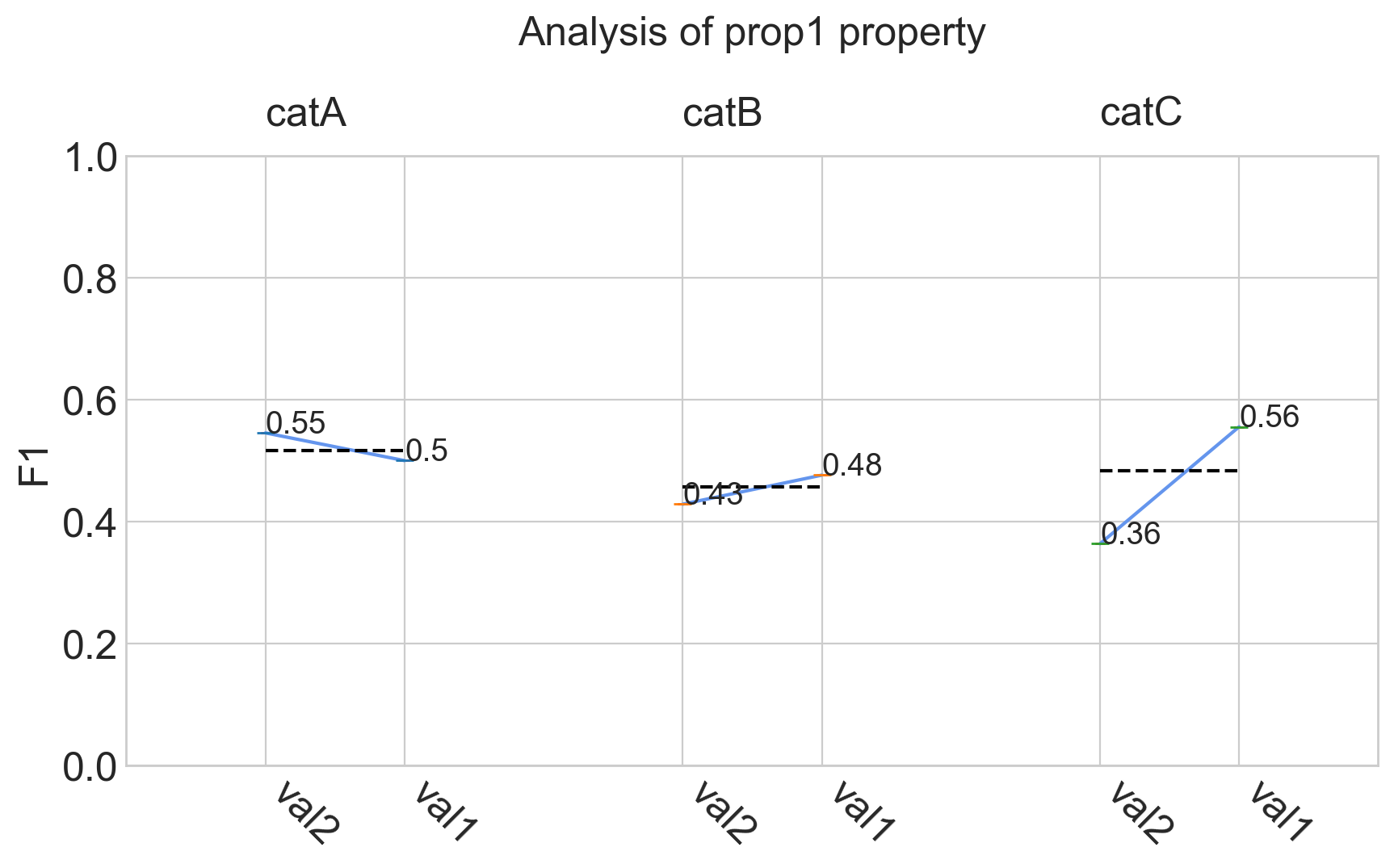## Models comparison

### Example

#### Classification

``````from odin.classes import ComparatorClassification

my_comparator = ComparatorClassification(dataset_gt_param, classification_type, models_proposals)
my_comparator.analyze_property('prop1')
``````

#### Localization

``````from odin.classes import ComparatorLocalization

my_comparator.analyze_property('prop1')
``````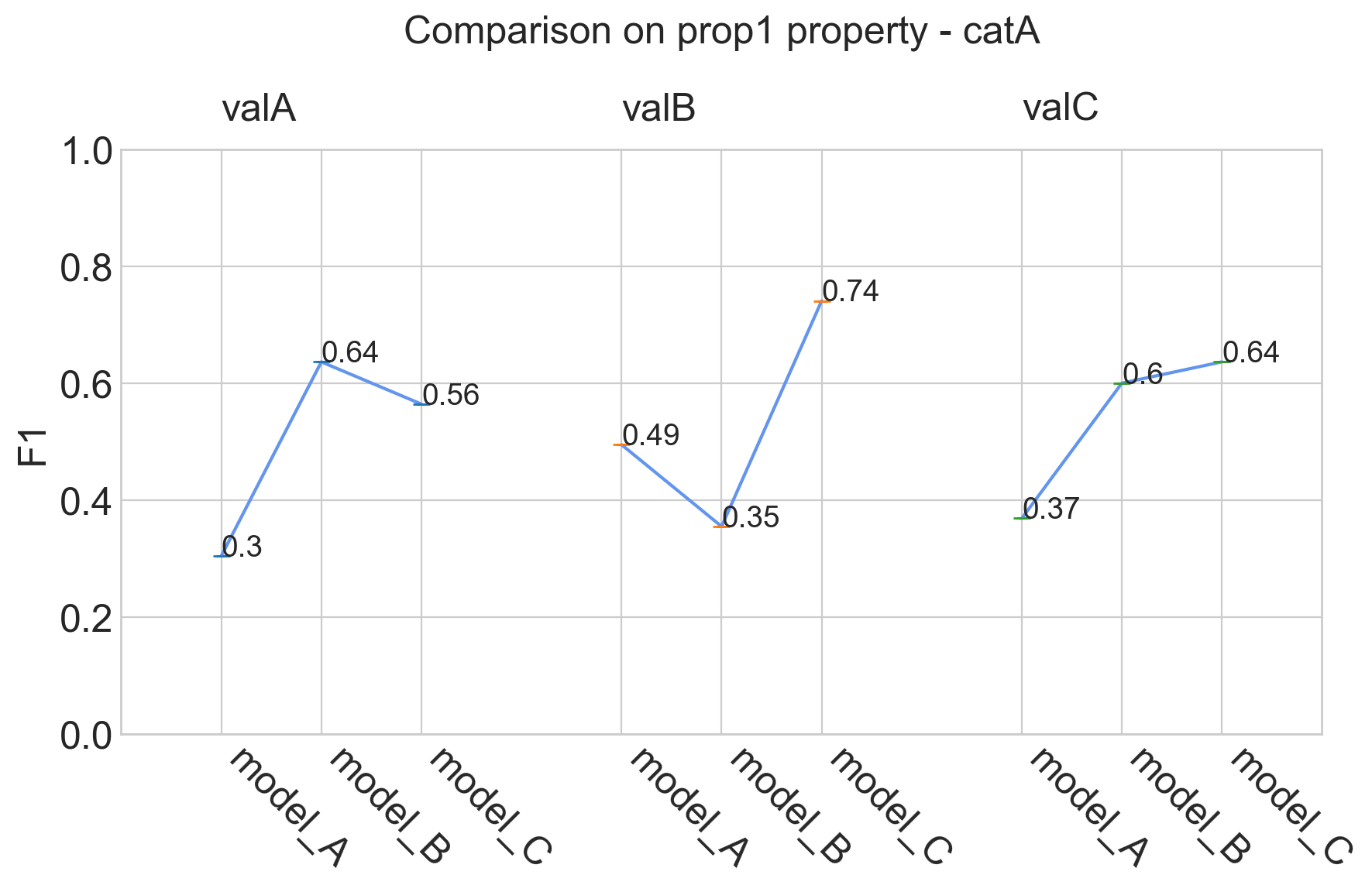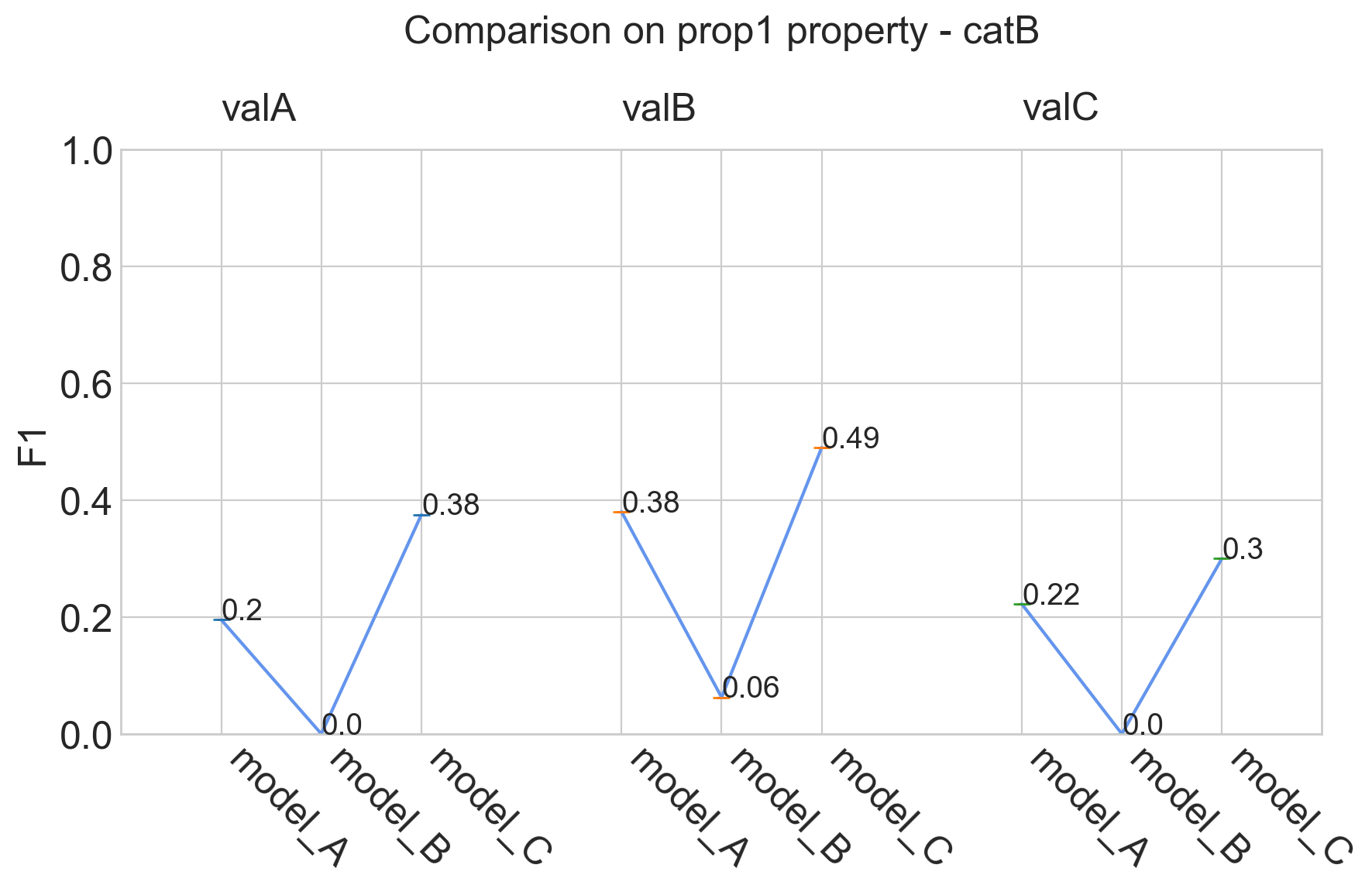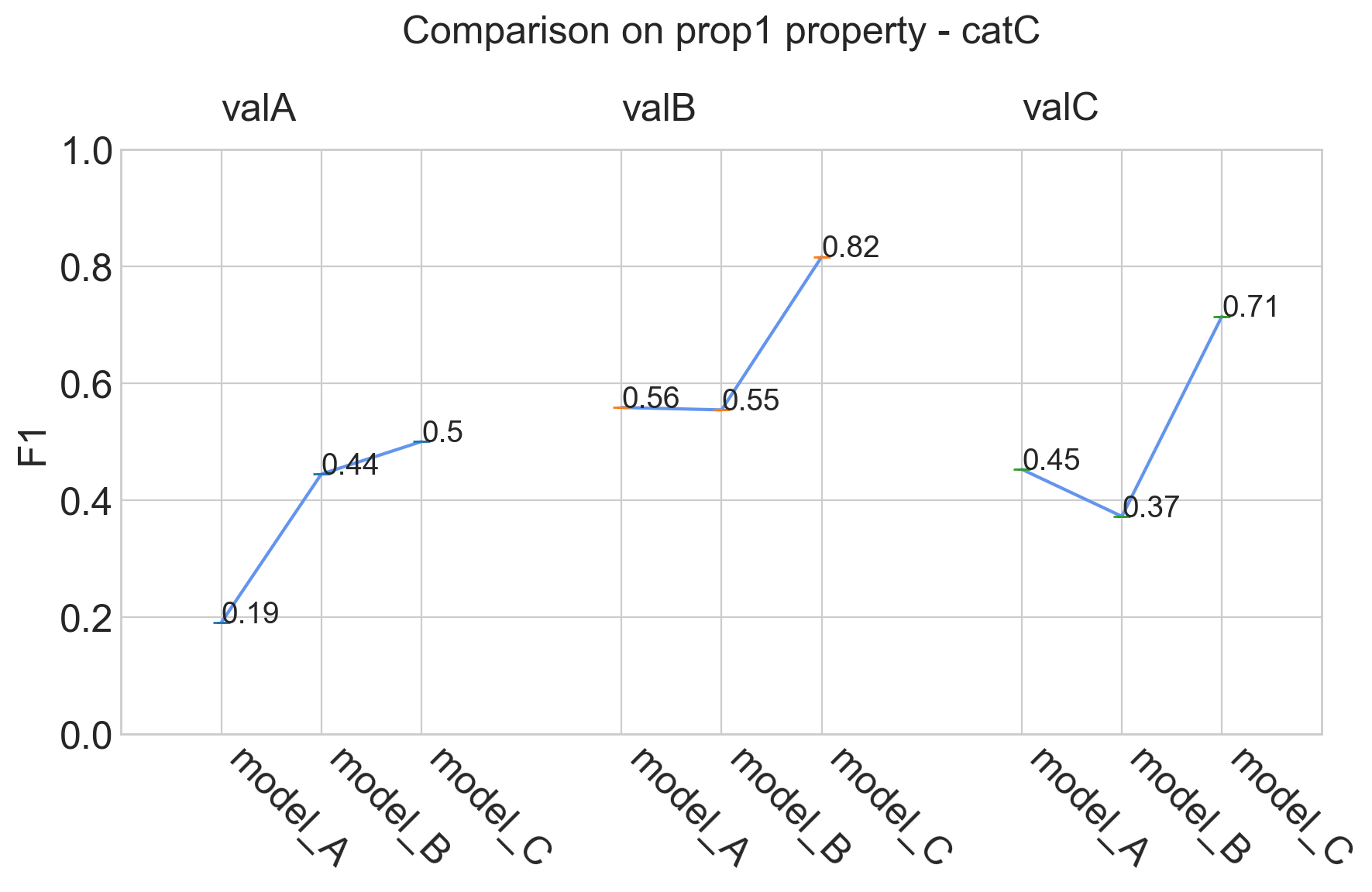Binary Classification Single-label Classification Multi-label Classification Object Detection Instance Segmentation
yes yes yes yes yes

# analyze_properties()

For each property, the model performances are analyzed for each category considering subsets of the data set which have a specific property value.

#### Parameters

properties

list, optional
List of properties to be included in the analysis. If not specified, all the properties are included.
(default is None)
categories

list, optional
List of categories to be included in the analysis. If not specified, all the categories are included.
(default is None)
metric

Metrics, optional
Evaluation metric used for the analysis. If not specified, the default one is used.
(default is None)
split_by

str, optional
If there are too many values, the plot is divided into subplots. The split can be performed by 'categories' or by 'meta-annotations'.
(default is 'meta-annotations')
show

bool, optional
Indicates whether the plot should be shown or not. If False, returns the results as dict.
(default is True)
sort

bool, optional
Indicates whether the property values should be sorted by the score achieved. Not supported for models comparisons.
(default is True)

### Example

#### Classification

``````from odin.classes import AnalyzerClassification

my_analyzer = AnalyzerClassification("my_classifier_name", my_classification_dataset)
my_analyzer.analyze_properties()
``````

#### Localization

``````from odin.classes import AnalyzerLocalization

my_analyzer = AnalyzerLocalization("my_detector_name", my_localization_dataset)
my_analyzer.analyze_properties()
``````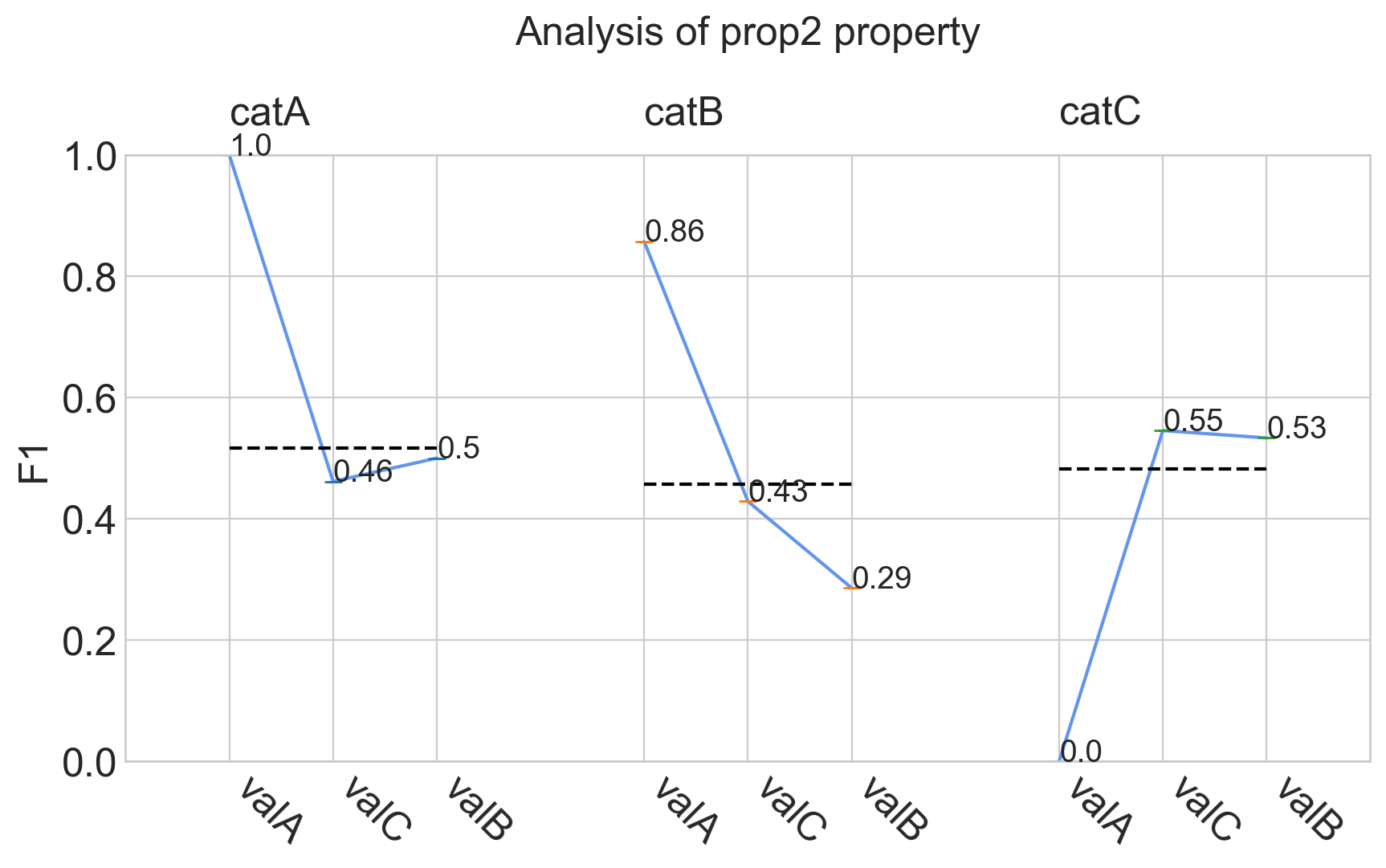Binary Classification Single-label Classification Multi-label Classification Object Detection Instance Segmentation
yes yes yes yes yes

# analyze_sensitivity_impact_of_properties()

It provides the sensitivity of the model for each property and the impact that the latter could have on the overall performance of the model. The sensitivity to a property is the difference between the maximum and minimum score obtained for that meta-annotation. The impact of a property, instead, is the difference between the maximum score achieved for it and the overall score obtained by the model.

#### Parameters

properties

list, optional
List of properties to be included in the analysis. If not specified, all the properties are included.
(default is None)
metric

Metrics, optional
Evaluation metric used for the analysis. If not specified, the default one is used.
(default is None)
show

bool, optional
Indicates whether the plot should be shown or not. If False, returns the results as dict.
(default is True)
sort

bool, optional
Indicates whether the properties should be sorted by the model sensitivity. Not supported for models comparisons.
(default is True)

### Example

#### Classification

``````from odin.classes import AnalyzerClassification

my_analyzer = AnalyzerClassification("my_classifier_name", my_classification_dataset)
my_analyzer.analyze_sensitivity_impact_of_properties()
``````

#### Localization

``````from odin.classes import AnalyzerLocalization

my_analyzer = AnalyzerLocalization("my_detector_name", my_localization_dataset)
my_analyzer.analyze_sensitivity_impact_of_properties()
``````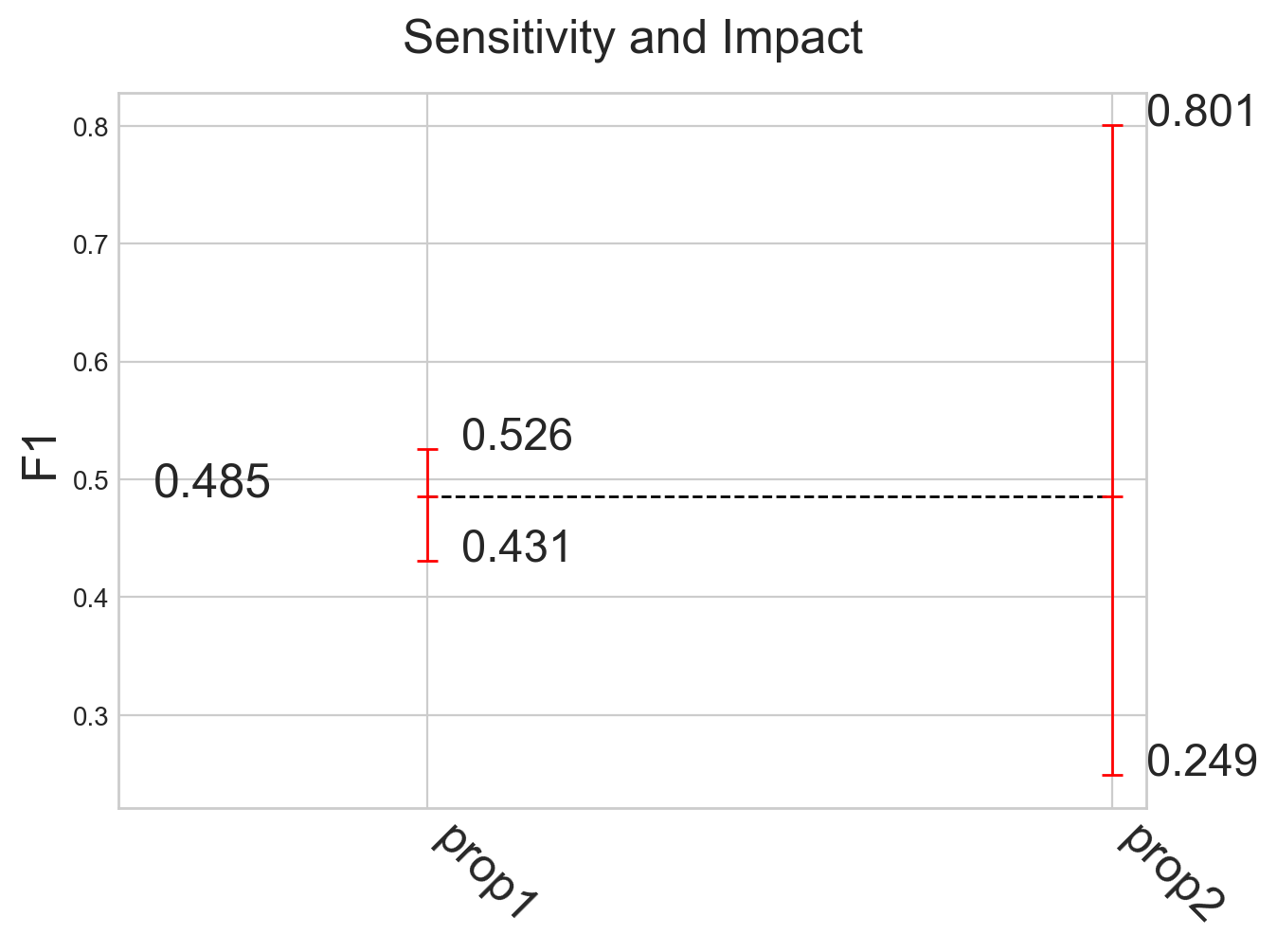## Models comparison

### Example

#### Classification

``````from odin.classes import ComparatorClassification

my_comparator = ComparatorClassification(dataset_gt_param, classification_type, models_proposals)
my_comparator.analyze_sensitivity_impact_of_properties()
``````

#### Localization

``````from odin.classes import ComparatorLocalization